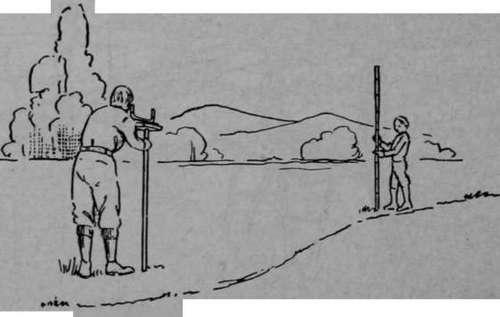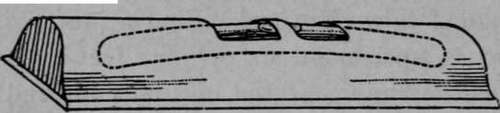An engineer's level is used to find the difference in level of two or more points (Fig. 63).

To practice using your level, find the difference in level of two points 100 feet apart on a road, sidewalk, or railroad.

To do this, you must first make what is called a leveling rod. Find a piece of wood about one or two inches square and six or more feet long, mark on it feet and inches, beginning at the bottom end, and your leveling rod is complete.Fig. 63. Homemade level in use

Now to find the difference in level of two points 100 feet apart, scratch a line or insert a small stake at one point, then pace off 100 feet and mark the second point. Now set up your level between the two points, ask a friend to hold the rod on the ground and upright, at the first point, sight along the water levels at the rod, and ask your friend to move his finger, or a white card, up and down until it is exactly in your line of sight. Now ask your friend to tell you exactly where his finger or card is and record this height. Let us suppose that it is 4 feet 6 inches above the ground. Now leave the level exactly where it is, ask your friend to hold the rod upright at the second point, and again sight along the water levels at the rod. Let us suppose that his finger or card is now exactly 3 feet above the ground.

The difference in level at the two points is 4 feet 6 inches minus 3 feet or 1 foot 6 inches. That is, the second point is 1 1/2 feet above the first point or the grade is 1.5 feet in 100, or 1.5 per cent.

You can now mark a third point 100 feet beyond the second point, set up your level between the second point and third point, place the rod at the second point, then at the third point, and find their difference in level as above. If the third point is 1 foot above the second, the total rise in the 200 feet is 2 1/2 feet; if, however, it is 1 foot below the second, the rise is 1 1/2 minus 1 or 1/2 foot in the 200 feet.

You can repeat this with as many points as you please.Fig. 64. A Spirit Level